# Ohms Law

Ohm's law shows a linear relationship between the voltage and the current in an electrical circuit.

The resistor's voltage drop and resistance set the DC current flow through the resistor.

With water flow analogy we can imagine the electric current as water current through pipe, the resistor as a thin pipe that limits the water flow, the voltage as height difference of the water that enables the water flow.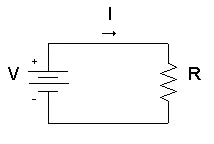## Ohm's law formula

The resistor's current I in amps (A) is equal to the resistor's voltage V in volts (V) divided by the resistance R in ohms (Ω):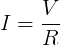V is the voltage drop of the resistor, measured in Volts (V). In some cases Ohm's law uses the letter E to represent voltage. E denotes electromotive force.

I is the electrical current flowing through the resistor, measured in  Amperes (A)

R is the resistance of the resistor, measured in Ohms (Ω)

#### Voltage calculation

When we know the current and resistance, we can calculate the voltage.

The voltage V in volts (V) is equal to the to the current I in amps (A) times the resistance R in ohms (Ω):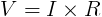#### Resistance calculation

When we know the voltage and the current, we can calculate the resistance.

The resistance R in ohms (Ω) is equal to the voltage V in volts (V) divided by the current I in amps (A):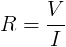Since the current is set by the values of the voltage and resistance, the Ohm's law formula can show that:

• If we increase the voltage, the current will increase.
• If we increase the resistance, the current will reduce.

#### Example #1

Find the current of an electrical circuit that has resistance of 50 Ohms and voltage supply of 5 Volts.

Solution:

V = 5V

R = 50Ω

I = V / R = 5V / 50Ω = 0.1A = 100mA

#### Example #2

Find the resistance of an electrical circuit that has voltage supply of 10 Volts and current of 5mA.

Solution:

V = 10V

I = 5mA = 0.005A

R = V / I = 10V / 0.005A = 2000Ω = 2kΩ

## Ohm's Law for AC Circuit

The load's current I in amps (A) is equal to the load's voltage VZ=V in volts (V) divided by the impedance Z in ohms (Ω):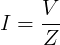V is the voltage drop on the load, measured in Volts (V)

I is the electrical current, measured in Amps (A)

Z is the impedance of the load, measured in Ohms (Ω)

#### Example #3

Find the current of an AC circuit, that has voltage supply of 110V∟70° and load of 0.5kΩ∟20°.

Solution:

V = 110V∟70°

Z = 0.5kΩ∟20° = 500Ω∟20°

I = V / Z = 110V∟70° / 500Ω∟20° = (110V / 500Ω) ∟ (70°-20°) = 0.22A ∟50°

### Ohm's Law Calculator (short form)

Ohm's law calculator: calculates the relation between Voltage, Current and Resistance.

Enter 2 values to get the third value and press the Calculatebutton:

 Enter Resistance: R = ohms (Ω) Enter Current: I = amps (A) Enter Voltage: V = volts (V)

Ohm's law calculator II ►

## Features of Ohms Law Calculator

Our Ohms Law Calculator allows the users to Calculate Ohms Law. Some of the prominent features of this utility are explained below.

#### No Registration

You don’t need to go through any registration process to use the Ohms Law Calculator. Using this utility, users to Calculate Ohms Law as many times as you want for free.

#### Fast conversion

This Ohms Law Calculator offers users the fastest Calculate. Once the user enters the  Ohms Law values ​​in the input field and clicks the Calculate button, the utility will start the conversion process and return the results immediately.

#### Saves Time and Effort

The manual procedure of Calculator Ohms Law isn’t an easy task. You must spend a lot of time and effort to complete this task. The Ohms Law Calculator allows you to complete the same task immediately. You will not be asked to follow manual procedures, as its automated algorithms will do the work for you.

##### Accuracy

Despite investing time and effort in manual Calculation, you might not be able to get your hands on accurate results. Not everyone is good at solving math problems, even if you think you're a pro, there's still a good chance you'll get in accurate results. This situation can be smartly handled with the help of a Ohms Law Calculator. You will be provided with 100% accurate results by this online tool.

##### Compatibility

The online Ohms Law converter perfectly works on all operating systems. Whether you have a Mac, iOS, Android, Windows, or Linux device, you can easily use this online Tool without facing any hassle.

###### 100% Free

You don't need to go through any registration process to use this Ohms Law Calculator. You can use this utility for free and do unlimited Ohms Law Calculate without any limitations.